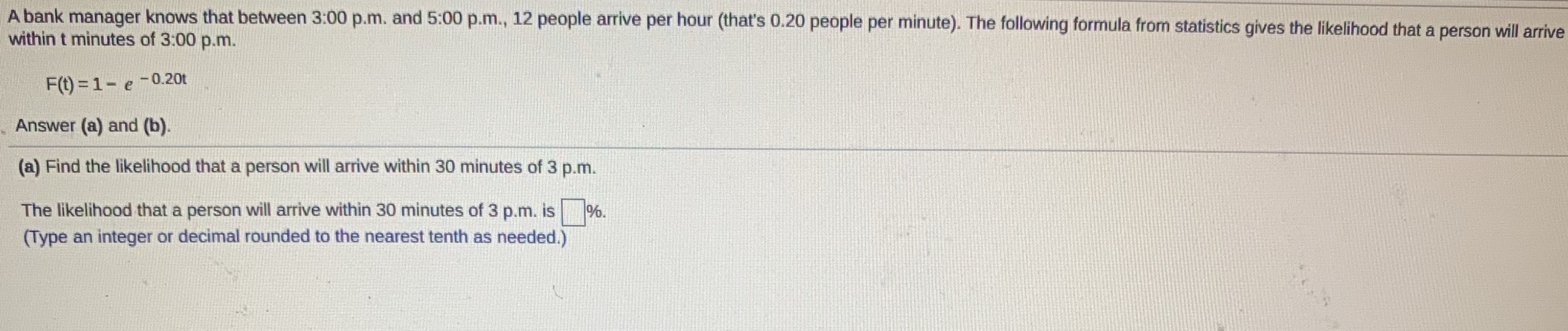### ¿Todavía tienes preguntas de matemáticas?

Pregunte a nuestros tutores expertos
Algebra
PreguntaA bank manager knows that between 3:00 p.m. and 5:00 p.m., $$12$$ people arrive per hour (that's $$0.20$$ people per minute). The following formula from statistics gives the likelihood that a person will arrive within $$t$$ minutes of $$3 : 00$$ p.m.

$$F ( t ) = 1 - e ^ { - 0.20 t }$$

(a) Find the likelihood that a person will arrive within $$30$$ minutes of $$3$$ p.m. The likelihood that a person will arrive within $$30$$ minutes of $$3 p . m$$ . is $$\square \%$$ . (Type an integer or decimal rounded to the nearest tenth as needed.)